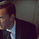# #sp500... X plays with Y in #cubes, & time repeats itself.

TVC:SPX   S&P 500
171 vues
Continuation of S&P 500 study,
X axis (time) and Y (price).

(A)
If we display the sections of
growth of the S&P 500 ,
by illustrating each period
of rising price, with a green square,
we get three (3) distinct periods
periods 1. and 2. and 3.

(B)
If we insert these squares 1. and 2.
inside the square of period 3. and that we
place the square of period 1. down and left
and the square of period 2. up and right,
as a result, in time and price, we note
the following unusual fact ...

(C)
In time, the period 1.
Plus the period 2.
Equals the period 3 ...
more or less, exactly.

(D)
In price, if we add the height
of period 1 plus the height
of period 2. we see that
the period 3. is higher than
the sum of periods 1 and 2.

François Normandeau
Directeur Régional (France)

static image:

## Commentairesahmetnmsl